Courses

# Thermodynamic Potential MCQ Level – 2

## 10 Questions MCQ Test Topic wise Tests for IIT JAM Physics | Thermodynamic Potential MCQ Level – 2

Description
This mock test of Thermodynamic Potential MCQ Level – 2 for IIT JAM helps you for every IIT JAM entrance exam. This contains 10 Multiple Choice Questions for IIT JAM Thermodynamic Potential MCQ Level – 2 (mcq) to study with solutions a complete question bank. The solved questions answers in this Thermodynamic Potential MCQ Level – 2 quiz give you a good mix of easy questions and tough questions. IIT JAM students definitely take this Thermodynamic Potential MCQ Level – 2 exercise for a better result in the exam. You can find other Thermodynamic Potential MCQ Level – 2 extra questions, long questions & short questions for IIT JAM on EduRev as well by searching above.
QUESTION: 1

### In a pressure cooker, the vapour pressure is greater than 1 atm. Under such condition, the boiling temperature of water will be Select one:

Solution:

From Claussius-Clapeyron eauation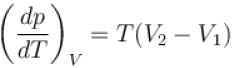on boiling V2 > V1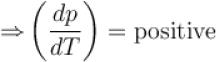Hence if we raise pressure above 1 atm the boiling point will also increase and will be higher than 373 K.
The correct answer is: Higher than 373 K

QUESTION: 2

### On a p-T graph, the curve which connects points at which vapour (v) and solid (s) exists in equilibrium called Select one:

Solution:

Sublimation curve connects points at which vapour and solid exists in equilibrium.
The correct answer is: Sublimation curve

QUESTION: 3

### In one of the Maxwell’s relationsequals Select one:

Solution:

From Maxwell’s equations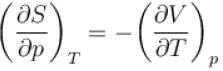The correct answer is: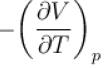QUESTION: 4

Entropy of a system having average energy E and partition function Z is defined as
Select one:

Solution:

F = –kT ln Z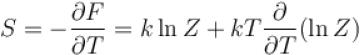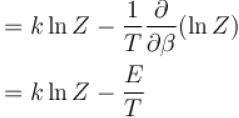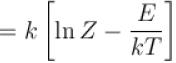= k[ln Z – βE]
The correct answer is: S = k[ln(Z) – βE]

QUESTION: 5

Helmholtz free energy, F = U – TS. Which relation holds true? (where U = Internal energy; S = Entropy)
Select one:

Solution:

F = U – TS
⇒ dF = dU – TdS – SdT
dF = –pdV – SdT ...(1)
take V constant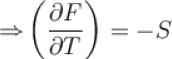take T constant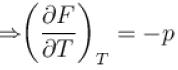The correct answer is: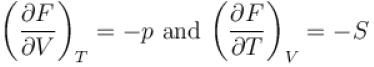QUESTION: 6

Which of the following is true with regards to critical point?
Select one:

Solution:

Critical point is that point on vapourization curve at which liquid and vapour phase have same density and above this temperature, a gas cannot be liquified by increasing the pressure.
The correct answer is: Above critical point, a gas cannot be liquified

QUESTION: 7

Helmholtz free energy function and internal energy U are related as
Select one:

Solution:

F = U – TS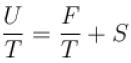differentiating w.r.t. ‘T’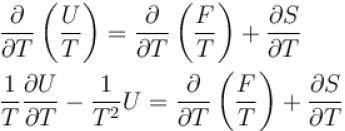keeping V constant, dW = 0
⇒dU = dQ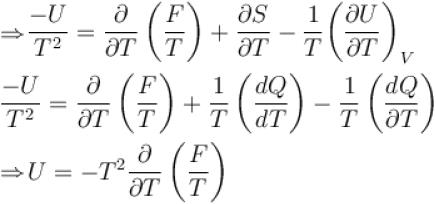The correct answer is: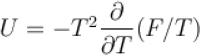QUESTION: 8

Which of the following relations between internal energy U and the canonical partition function Z is true?
Select one:

Solution:

Internal energy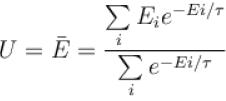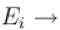Energy of the system in the ith state the partition function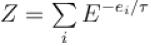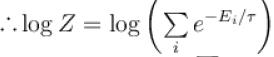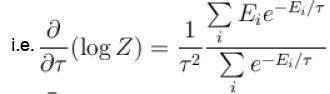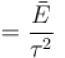Thus,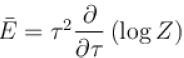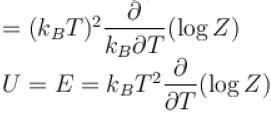The correct answer is: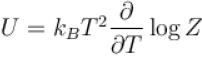QUESTION: 9

Consider two level question system with energies ε1 = 0, ε2 = ε, The Helmholtz free energy of the system is given by
Select one:

Solution: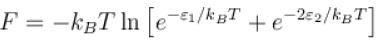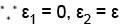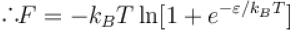The correct answer is: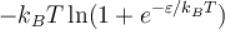QUESTION: 10

The boiling point of water will decrease as we go to a higher altitude because
Select one:

Solution:

As we go to a higher altitude, the pressure decreases and from ClaussiusClapeyron equation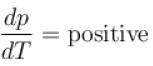Since dp is negative dT has to be negative hence boiling point decreases.
The correct answer is: Pressure decreases